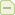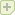#### 连连看 游戏分析与制作　源码下载

Flash游戏教程 2007-10-31 14:16

国庆前后完成了连连看的分析和代码实践，但一直没有精力来完成分析文章，现在先把这个基本定稿的测试版代码发出来，有兴趣的朋友先看看，回头再补上分析过程。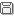压缩包下载

大致的分析思路是这样：

１、图块的摆放的设定。

２、鼠标操作的流程结构，请参考我以前写过的　配对游戏　记忆力游戏分析文章。

３、位置关系的检测。分四种: a、直线连接　b、直角连接（具有一个拐点）　c、S形连接（具有两个拐点）　d、　C形连接（具有两个拐点）。　在检测时，分别对这四种情况进行检测。

４、关卡的设定，利用数组指定好每一关的行列个数。通过游戏的初始化函数进行参数化的摆放。

function chk_S_Line(ar:Array, a:MovieClip, b:MovieClip):Boolean {
//内部S形二次拐点的判定
//先横向搜索
//if (Math.abs(a.c - b.c) > 1) {

//在进行S 形判断之前必须先进行直线连通的判断, 以确保两个MC不在同一行或同一列上. 本条if 语句是比较的多余,只是为了确保两个MC块之间有足够的空间进行S 形连接, 实际上通过 for 循环的控制条件同样可以达到这个目的.

// S 形连接的画线效果比L 形画线漂亮, 在同等连通条件下,先做哪个检测,就可以得到哪种连接. 我的例子中,先做的是L 形的检测,所以一次拐点优先连通.

//此时,先按列号的位置进行横向的搜索, 搜索的方向是,先从列号较小的块出发,向第一个拐点进行连通测试,再从第一拐点至第二拐点,再从第二拐点至第二个块. 在for 循环中,可以看到,由两个拐点构成的竖线是从左向右逐行移动检测的.

var begin, end:Number;
a.c < b.c ? (begin = a.c, end = b.c) : (begin = b.c, end = a.c);
for (var i = begin + 1; i < end; i++) {
if (chkP2P(ar, ar[a.r][i], a) && chkP2P(ar, ar[b.r][i], b) && chkP2P(ar, ar[a.r][i], ar[b.r][i]) && !ar[a.r][i]._visible && !ar[b.r][i]._visible) {

//判断条件中,三条线段必须是连通的, 两个拐点必须是不可见的( 表示连通 ). 当这些条件都符合时,记录连通点的对象,并且函数返回 true.
pa = a;
pb = b;
pc = ar[b.r][i];
pd = ar[a.r][i];
return true;
}
}
// }
//再纵向搜索
//if (Math.abs(a.r - b.r) > 1) {//  这个判断条件同样是多余的, 后面的for 循环可以自行得到正确的范围.
var begin, end:Number;
a.r < b.r ? (begin = a.r, end = b.r) : (begin = b.r, end = a.r);
for (var i = begin + 1; i < end; i++) {//  在这个for 循环中,两个捌点构成的横线是从上向下逐行移动检测的.
if (chkP2P(ar, ar[i][a.c], a) && chkP2P(ar, ar[i][b.c], b) && chkP2P(ar, ar[i][a.c], ar[i][b.c]) && !ar[i][a.c]._visible && !ar[i][b.c]._visible) {
pa = a;
pb = b;
pc = ar[i][b.c];
pd = ar[i][a.c];
return true;
}
}
//}
return false;
}
function chk_C_Line(ar:Array, a:MovieClip, b:MovieClip):Boolean {
//C形U 形连通二次拐点的判定。
//为获取最短路径，从a、b图片行列号所构成的方形区域向外逐层搜索，若有通路必为最短路径。
var cLeft, cRight, rTop, rBottom:Number;//边界信息
a.c <= b.c ? (cLeft = a.c - 1, cRight = b.c + 1) : (cLeft = b.c - 1, cRight = a.c + 1);
a.r <= b.r ? (rTop = a.r - 1, rBottom = b.r + 1) : (rTop = b.r - 1, rBottom = a.r + 1);

while (cLeft >= 0 || cRight < colNum || rTop >= 0 || rBottom < rowNum) { // 只要有一个方向上未到达检测的边界,循环就会继续,  colNum rowNum 是全局变量,用来记录数组的最大行号和最大列号, 实际上可以用 ar.length  ar.length 来分别替代.用全局变量更直观一些.
//若通过左侧 [　形连接可以连通，则返回真。
if (cLeft >= 0) {
if (chkP2P(ar, ar[a.r][cLeft], a) && chkP2P(ar, ar[b.r][cLeft], b) && chkP2P(ar, ar[a.r][cLeft], ar[b.r][cLeft]) && ar[a.r][cLeft]._visible == false && ar[b.r][cLeft]._visible == false) {
pa = a;
pb = b;
pc = ar[b.r][cLeft];
pd = ar[a.r][cLeft];
return true;
}
}
//若通过右侧 ]　形连接可以连通，则返回真。
if (cRight < colNum) {
if (chkP2P(ar, ar[a.r][cRight], a) && chkP2P(ar, ar[b.r][cRight], b) && chkP2P(ar, ar[a.r][cRight], ar[b.r][cRight]) && ar[a.r][cRight]._visible == false && ar[b.r][cRight]._visible == false) {
pa = a;
pb = b;
pc = ar[b.r][cRight];
pd = ar[a.r][cRight];
return true;
}
}
//若通过上部 n　形连接可以连通，则返回真。
if (rTop >= 0) {
if (chkP2P(ar, ar[rTop][a.c], a) && chkP2P(ar, ar[rTop][b.c], b) && chkP2P(ar, ar[rTop][a.c], ar[rTop][b.c]) && ar[rTop][a.c]._visible == false && ar[rTop][b.c]._visible == false) {
pa = a;
pb = b;
pc = ar[rTop][b.c];
pd = ar[rTop][a.c];
return true;
}
}
//若通过下部 U　形连接可以连通，则返回真。
if (rBottom < rowNum) {
if (chkP2P(ar, ar[rBottom][a.c], a) && chkP2P(ar, ar[rBottom][b.c], b) && chkP2P(ar, ar[rBottom][a.c], ar[rBottom][b.c]) && ar[rBottom][a.c]._visible == false && ar[rBottom][b.c]._visible == false) {
pa = a;
pb = b;
pc = ar[rBottom][b.c];
pd = ar[rBottom][a.c];
return true;
}
}
cLeft--, cRight++, rTop--, rBottom++; //  在循环的最后, 让边界判断变量向外"扩展", 扩张出实际数组范围也不用担心,因为前面有if 语句来把关, 超出范围的边界不在检测范围内. 这样能够确保得到最短路径. 当 左侧 和右侧同时可以进行C形连接时,我这里优先选择的是左侧, 这是和检测顺序有关, 得到的都是最短路径.
}
return false;
}

《推箱子》系列教程：
原创教程《推箱子游戏的分析》

《推箱子游戏的分析》续

电击方块游戏的分析

按键平滑移动的代码方案。{commentauthor}
{commenttime}
{commentnum}
{commentcontent}
###### 作者:
{commentrecontent}
﻿

#### 注册5D通行证,立刻拥有自己的博客Join 5D!发表留言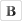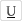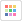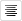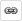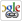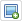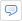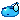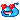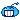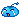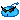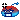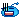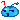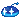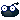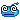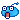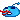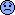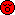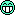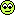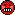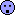禁止笑脸转换 No smiley